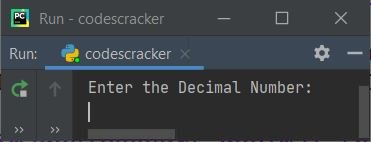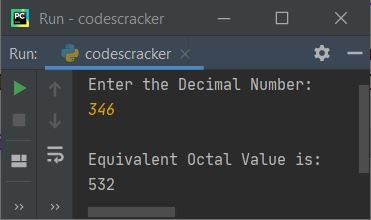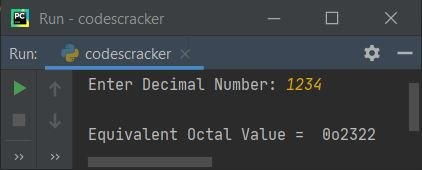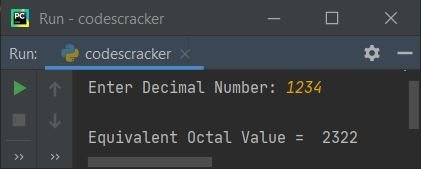# Python Program to Convert Decimal to Octal

This article is created to cover some programs in Python, to convert decimal number entered by user at run-time to its equivalent octal value. Here are the list of programs:

• Decimal to Octal using list and while Loop
• Using oct() method
• Using function
• Using class

Note - Before creating these programs, if you're not aware about steps used for the conversion, then refer to Decimal to Octal Conversion Steps and Example to get every required things about the topic.

## Decimal to Octal using List and while Loop

To convert decimal to octal number in Python, you have to ask from user to enter a number in decimal number system, then convert that number into its equivalent value in octal number system as shown in the program given below. The question is, write a Python program to convert decimal to octal using list and while loop. Here is its answer:

```print("Enter the Decimal Number: ")
decnum = int(input())

i = 0
octnum = []
while decnum!=0:
rem = decnum%8
octnum.insert(i, rem)
i = i+1
decnum = int(decnum/8)

print("\nEquivalent Octal Value is: ")
i = i-1
while i>=0:
print(octnum[i], end="")
i = i-1
print()```

Here is its sample run:Now supply the input say 346 as a decimal number, then press `ENTER` key to convert and print its equivalent octal value as shown in the snapshot given below:The dry run of above program with user input 346 goes like:

• Initial values, decnum=346 (entered by user), i=0
• The condition (of while loop) decnum!=0 or 346!=0 evaluates to be true, therefore program flow goes inside the loop
• decnum%8 or 346%8 or 2 gets initialized to rem
• And using the following statement:
`octnum.insert(i, rem)`
the value of rem gets initialized to octnum[i]. So octnum[i] or octnum = 2
• i+1 or 0+1 or 1 gets initialized to i
• int(decnum/8) or int(346/8) or 43 gets initialized to decnum
• The condition of while loop again gets evaluated with new value of decnum
• That is, the condition decnum!=0 or 43!=0 evaluates to be true again, therefore program flow goes inside the loop. This process continues until the condition evaluates to be false
• In this way, the list octnum holds equivalent octal value of given decimal number by user at run-time
• After exiting from the loop, decrement the value of i. Because before the condition of while loop evaluates to be false, the value of i was incremented
• Now print octnum[] list one by one from its last index to 0th index

#### Modified Version of Previous Program

This is the modified version of previous program. This program uses end= to skip printing of an automatic newline using print()

```print("Enter Decimal Number: ", end="")
dnum = int(input())

i = 0
onum = []
while dnum!=0:
onum.insert(i, dnum % 8)
i = i+1
dnum = int(dnum / 8)

print("\nEquivalent Octal Value = ", end="")
i = i-1
while i>=0:
print(onum[i], end="")
i = i-1

print()```

Here is its sample run with user input 3112 as decimal number input:## Decimal to Octal using oct()

The method oct() returns octal equivalent of value passed as its argument.

```print("Enter Decimal Number: ", end="")
dnum = int(input())

onum = oct(dnum)
print("\nEquivalent Octal Value = ", onum)```

Here is its sample run with decimal number input as 1234:Note - To skip first two characters of octal number output, add [2:] after onum while printing. That is, replace the following statement (from above program):

`print("\nEquivalent Octal Value = ", onum)`

with the statement given below:

`print("\nEquivalent Octal Value = ", onum[2:])`

Now the output looks like:## Decimal to Octal using Function

This program is created using user-defined and predefined functions both. The user-defined function named DecToOct() receives a value as its argument and returns its octal equivalent using oct() method.

```def DecToOct(d):
return oct(d)

print("Enter Decimal Number: ", end="")
dnum = int(input())

onum = DecToOct(dnum)
print("\nEquivalent Octal Value =", onum[2:])```

Using the following statement:

`onum = DecToOct(dnum)`

The value of dnum variable (stores decimal number entered by user) gets passed to DecToOct() function. And this function returns its equivalent octal value. Therefore this value (octal) gets returned and initialized to onum variable. Now print the value of onum variable as octal equivalent of given decimal number.

## Decimal to Octal using Class

This program is created using class, an object-oriented feature of Python. That is, a class named CodesCracker is created with a member function named DecToOct(). To access member function of a class, an object of class can be used. Therefore an object named obj is created of class CodesCracker to access its member function named DecToOct() using dot (.) operator.

```class CodesCracker:
def DecToOct(self, d):
return oct(d)

print("Enter Decimal Number: ", end="")
dnum = int(input())

obj = CodesCracker()
onum = obj.DecToOct(dnum)
print("\nEquivalent Octal Value =", onum[2:])```

#### Same Program in Other Languages

Python Online Test

« Previous Program Next Program »